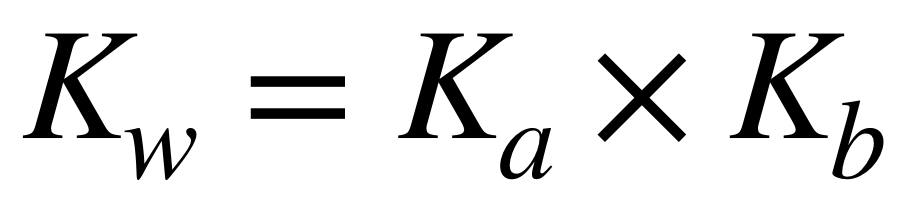# Problem: Given that Ka for HIO is 3.2 × 10-11 at 25°C, what is the value of Kb for IO– at 25°C?

###### FREE Expert Solution

Recall: The Ka of an acid and the Kb of its conjugate base are related to each other by the following equation:where Kw is the autoionization constant for water. At 25˚C, Kw = 1.0 x 10–14.###### Problem Details

Given that Ka for HIO is 3.2 × 10-11 at 25°C, what is the value of Kb for IO at 25°C?